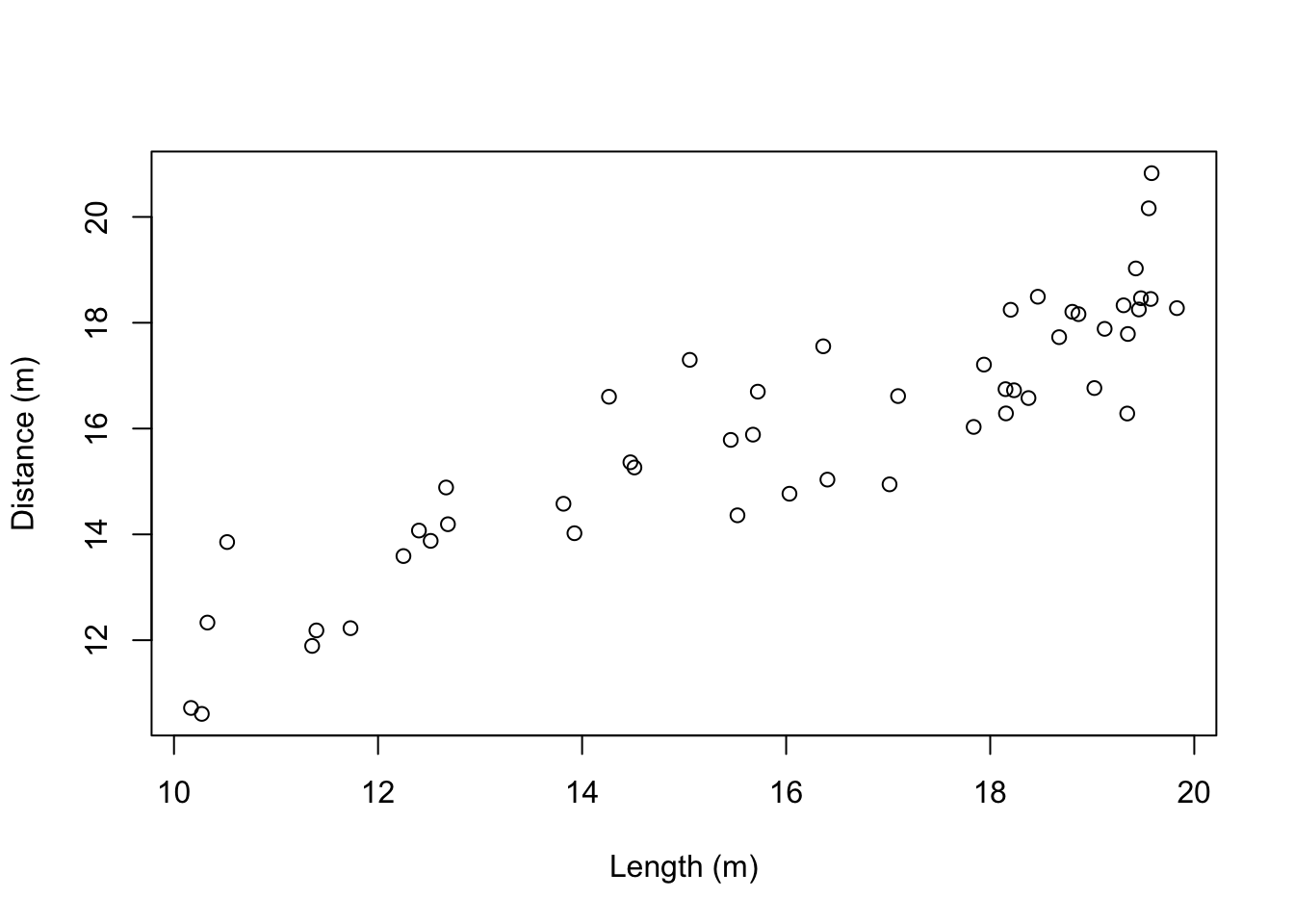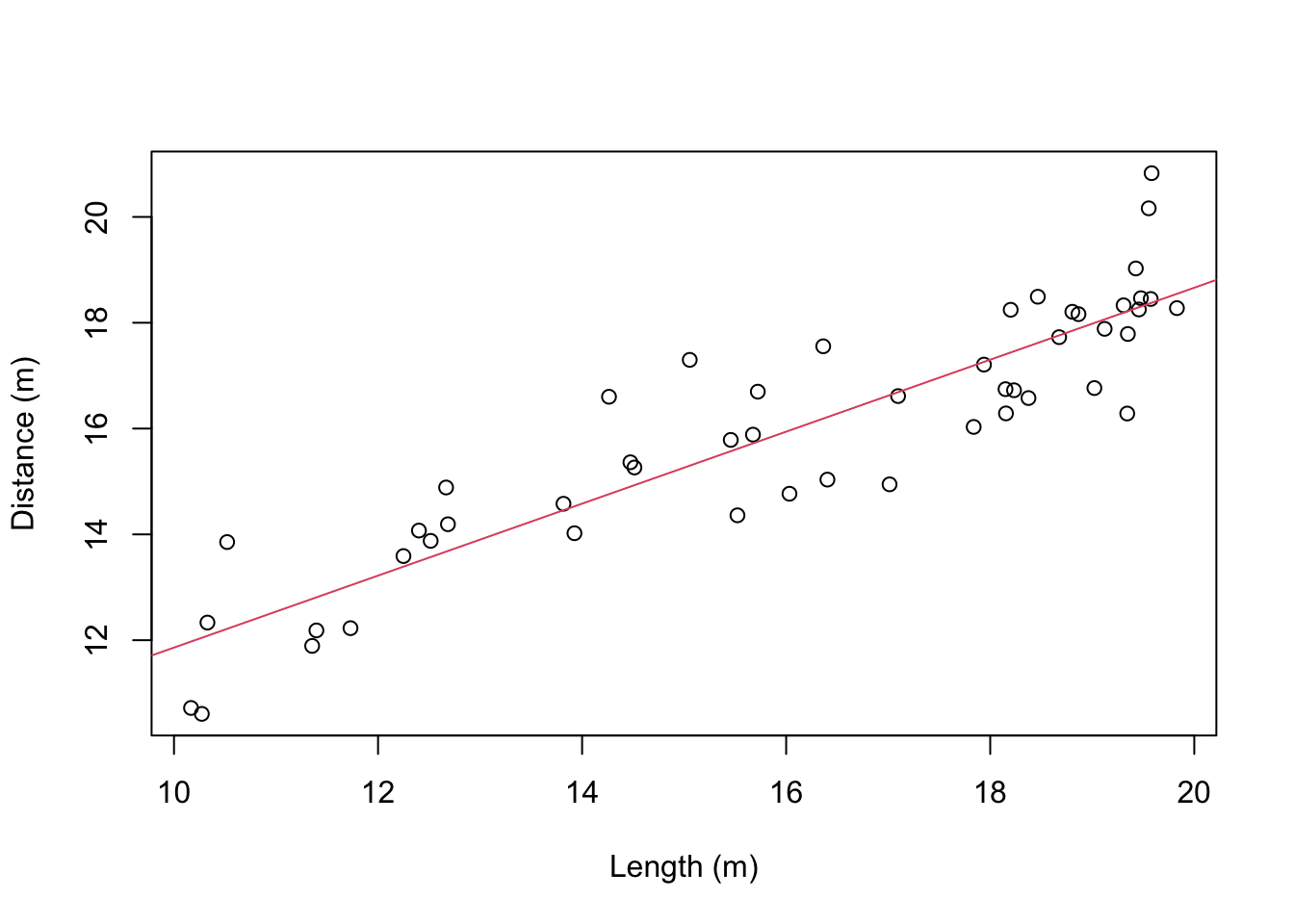Due midnight 18th February 20221

## Question 1: Dragonfire

As part of our investigation of the re-introduction of dragons into Trondheim, we have been looking at fire precautions. We sent a student out to measure the lengths of 50 dragons, and (from a safe distance) recorded how far they could breath fire after being poked with a very long stick. Each dragon was only tested once. Note that no major cities were destroyed during this experiment.

This is the data:

DragonFire <- read.csv(file="https://www.math.ntnu.no/emner/ST2304/2021v/Week05/DragonFire.csv")

plot(DragonFire$Size, DragonFire$Distance, xlab="Length (m)", ylab="Distance (m)")It looks like there is a linear relationship between distance and the length of a dragon. We can fit a linear model, that looks like:

$y = \alpha + \beta x + \epsilon$ ß Remember, we are using a maximum likelihood approach to calculate estimates for the parameters $$\alpha$$ (the intercept) and $$\beta$$ (the slope). That means we are looking for estimates that have the highest probability of having produced the data (maximum likelihood estimators; MLEs).

1. Fit a linear model to the Dragon Fire data using R and get the coefficient estimates (MLEs for the intercept and slope)

mod <- lm(Distance~Size, data=DragonFire)
coef(mod)

How certain can we be about the estimates? We can answer this by calculating confidence intervals. Remember the definition of a confidence interval:

If we repeat our sample many times, 95% of the confidence intervals drawn would contain the true population value

So, we assume the MLEs represent the true parameter values, and every time we repeat the sampling of our data, we can calculate slightly different MLEs. Lets inspect fit of the model visually by adding a mean line to the scatter plot:

plot(DragonFire$Size, DragonFire$Distance, xlab="Length (m)", ylab="Distance (m)")
abline(lm(Distance~Size, data=DragonFire), col=2) # add mean lineIf we retrieve estimates from a model every time we sample data, we get a distribution of the slope estimator. We can do this artificially by simulation. For that, we have to assume that we know the true values of the parameters. First we have a function to simulate the data that takes the true parameter values as input, and a second function that calculates an estimate of the slope from the simulated data.

alpha.hat <- coef(mod)
beta.hat <- coef(mod)
sigma.hat <- summary(mod)$sigma # std deviation # function to simulate the data, fit the model & return the slope estimate SimData <- function(alpha, beta, sigma, X) { E.y <- alpha + beta*X # calculate the line y.sim <- rnorm(length(X), E.y, sigma) # simulate the data model <- lm(y.sim ~ X) # fit the model coef(model) # return the estimated slope } # Run one simulation, to check the function works SimData(alpha=alpha.hat, beta=beta.hat, sigma=sigma.hat, X=DragonFire$Size)
##         X
## 0.7526991

You should read the function to check that you understand what each line does, and what the function produces. For example, the code coef(model) takes the second element of the object of coefficients from the model. This is the estimate of the slope value for the linear regression.

With this function, we can “sample” the data lots of times, and get a distribution for the estimator of the slope:

1.1. Run the code, plot the histogram, and use quantile() to find the 95% confidence interval for the MLE of the slope parameter.

Rep.beta <- replicate(1000, SimData(alpha=alpha.hat,
beta=beta.hat,
sigma=sigma.hat,
X=DragonFire$Size)) hist(Rep.beta) # Calculate the 95% confidence interval quantile(Rep.beta, c(0.025, 0.975)) This is the distribution of the estimates of the slope that would come if the data were repeatedly sampled. The mean of this distribution should be similar to the value of the “true” parameter: Rep.beta <- replicate(1000, SimData(alpha=alpha.hat, beta=beta.hat, sigma=sigma.hat, X=DragonFire$Size))

mean(Rep.beta) # Mean of sampling distribution
##  0.6825849
beta.hat # "True" parameter used in simulation
##      Size
## 0.6804651

The confidence intervals are a way of summarising the whole distribution: they put limits on the most likely values.

R can also calculate the confidence interval itself using the confint() function.

1.2. Use confint() to calculate the confidence interval for your Dragon Fire model. Is it the same/similar/different to the one you got by simulation? Why?

1.3 Discuss the interpretation of the confidence interval. Explain exactly what it means.

Now we can do some prediction. One released dragon has taken residence in a small lake, and it will sometimes sit at the water’s edge and breath fire. We want to know what distance away from the lake is safe, and for example where should trees be cut down so they aren’t burnt down. We know this dragon is 12m long, so we want to predict how far it can breathe fire.
We do this using the model:

$y_{pred} = \hat{\alpha} + \hat{\beta} x$ where $$\hat{\alpha}$$ and $$\hat{\beta}$$ are the MLEs, and $$x$$ is the value we want to predict for.

1.4. calculate the predicted value of $$y$$ with $$x=12$$ and the MLEs for the parameters. Use the equation above and fill in the MLEs you calculated in qu 1.

We can summarise our uncertainty in the prediction with more confidence intervals.

The function below is a modification of the function above: this one simulates the prediction. By simulate, we mean that this mimics what would happen if we repeated our sampling (to get our data) many many times and each time made a prediction. In reality, we don’t do this, but we use the maths involved in maximum likelihood estimation to represent this. R will do this with mathematics.

1.5. simulate 1000 predictions for $$x=12$$ and calculate the confidence interval for the prediction. You can use the code below but you will need to edit it to predict for the right $$x$$ value.

# function to simulate the data, fit the model & return a prediction
SimPrediction <- function(alpha, beta, sigma, X, x.pred) {
E.y <- alpha + beta*X
y.sim <- rnorm(length(X), E.y, sigma) # simulates values of y
model <- lm(y.sim ~ X) # run model on this simulated data
pred.mu <- coef(model) + coef(model)*x.pred # mean prediction
pred.mu
}

# Run one simulation, to check the function works
SimPrediction(alpha=alpha.hat,
beta=beta.hat,
sigma=sigma.hat,
X=DragonFire$Size, x.pred=1) ## (Intercept) ## 6.126877 # use the SimPrediction function to predict for 1000 different data sets # make sure to change x.pred to the value of x you want to predict for Rep.pred <- replicate(1000, SimPrediction(alpha=alpha.hat, beta=beta.hat, sigma=sigma.hat, X=DragonFire$Size,
x.pred=20))

hist(Rep.pred)

# Calculate the 95% confidence interval of the predictions
quantile(Rep.pred, c(0.025, 0.975))

Above you simulated new repeating sampling of dragon fire, fitting a model to each new sample, and each time using the model to make a prediction.

Below, is a very similar function. But in this function there is an extra process included, new <- rnorm(length(x.pred), pred.mu, sigma). This second function should more closely represent what would happen if repeated the dragon fire sampling many times, fit the model each time, and then went to collect one more sample for a given size of dragon e.g. $$x$$ = 12.

1.6. in this second function we have to use rnorm() twice. Why is it used a second time (in new <- rnorm(length(x.pred), pred.mu, sigma))? What are we calculating if we don’t do that i.e. in the first function? (hint: what are the different sources of uncertainty in predictions?)

1.7. simulate 1000 predictions for $$x=12$$ using the SimPrediction2 function and calculate the confidence interval for these predictions. How is this different to the confidence interval in 1.5?

1.8. the confidence interval from 1.7 more accurately represents a 95% prediction interval (the confidence interval for a prediction) how would you interpret these confidence intervals for the prediction? i.e. give a definition of what they mean/represent.

# function to simulate the data, fit the model & return a prediction
SimPrediction2 <- function(alpha, beta, sigma, X, x.pred) {
E.y <- alpha + beta*X
y.sim <- rnorm(length(X), E.y, sigma) # simulates values of y
model <- lm(y.sim ~ X) # run model on this simulated data
pred.mu <- coef(model) + coef(model)*x.pred # mean prediction
new <- rnorm(length(x.pred), pred.mu, sigma) # simulated new observations
new
}

# Run one simulation, to check the function works
SimPrediction2(alpha=alpha.hat,
beta=beta.hat,
sigma=sigma.hat,
X=DragonFire$Size, x.pred=1) ##  5.484033 Rep.pred2 <- replicate(1000, SimPrediction2(alpha=alpha.hat, beta=beta.hat, sigma=sigma.hat, X=DragonFire$Size,
x.pred=20))

hist(Rep.pred2)

# Calculate the 95% interval of the data
quantile(Rep.pred2, c(0.025, 0.975))

R can also calculate the prediction itself using the predict() function:

# create new data to predict for
PredictionData <- data.frame(Size=20)
# get a point prediction
predict(mod, newdata = PredictionData)
# get a point prediction plus prediction interval
predict(mod, newdata = PredictionData, interval="prediction")

1.9. Try out predict(mod, newdata = PredictionData, interval="confidence") instead of predict(mod, newdata = PredictionData, interval="prediction"). What is different about these two types of prediction. (Hint: compare these to the simulations in qu 1.5-1.8).

1.10. What distance away from the lake would YOU consider safe from dragon fire? Why is the predicted distance not a good suggestion? Explain your answer.

## Question 2: How long will the Deathly Hallows be?

Here we have the lengths of the Harry Potter book plotted against their order. This analysis was originally done in 2006, where the question was how long the next book would be.

First, you can just run the following code, to create the data and plot it.

# Make the data frame using function data.frame()
# each name before the = is the name of a column
# the elements after the = are the values for that column
HPbooks <- data.frame(
Book = c("Philosopher's Stone", "Chamber of Secrets", "Prisoner of Azkaban",
"Goblet of Fire", "Order of the Phoenix", "Half-Blood Prince",
"Deathly Hallows"),
Length = c(223, 251, 317, 636, 766, 607, NA), # 607
Date = c(1997, 1998, 1999, 2000, 2003, 2005, 2007)
)

HPbooks$BookOrder <- 1:nrow(HPbooks) # nrow() = count number of rows # This will make the book titles easier to read # Feel free to ignore this: you don't need to know about gsub() for this course HPbooks$BookLabels <- gsub("Harry Potter and the ", "", HPbooks$Book) # set margins of the plot par(mar=c(6.1,4.1,1,1)) plot(HPbooks$BookOrder, HPbooks$Length, xlab="", ylab="Number of pages", xaxt="n") # xaxt = "n" tells R not to include an x-axis # instead we add it manually axis(1, at=HPbooks$BookOrder, padj=0.8, cex.axis=0.8,
labels = gsub(" ", "\n", HPbooks\$BookLabels))
text(7, 600, "?", col=2, cex=5)